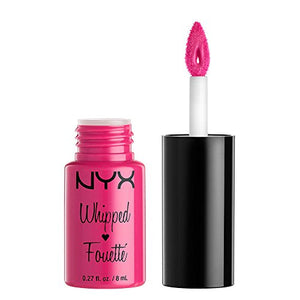# Nyx Whipped Lip & Cheek Souffle, Pink Lace, Wlcs08, 0.27 Fl. Oz.

• \$21.58
• Save \$15.60

• O

• u

• r

• t

• w

• o

• -

• i

• n

• -

• o

• n

• e

• m

• o

• u

• s

• s

• e

• c

• a

• n

• b

• e

• u

• s

• e

• d

• t

• o

• p

• r

• e

• c

• i

• s

• e

• l

• y

• l

• i

• n

• e

• l

• i

• p

• s

• a

• n

• d

• o

• n

• t

• h

• e

• a

• p

• p

• l

• e

• s

• o

• f

• y

• o

• u

• r

• c

• h

• e

• e

• k

• s

• f

• o

• r

• a

• s

• o

• f

• t

• ,

• n

• a

• t

• u

• r

• a

• l

• f

• l

• u

• s

• h

Indulge your cravings with our Whipped Lip & Cheek Souffl. Our two-in-one mousse can be used to precisely line lips and on the apples of your cheeks for a soft, natural flush. Achieve a delicious wash of color with a matte finish using this creamy and multipurpose formula.

every pages